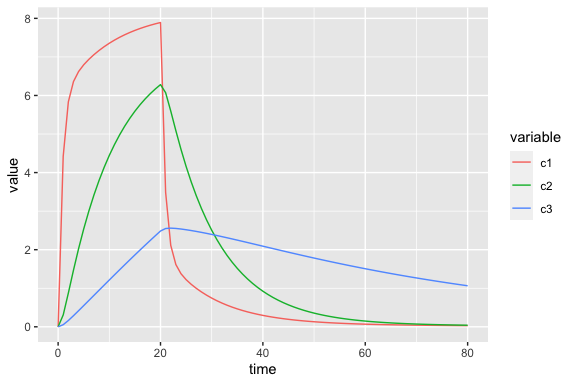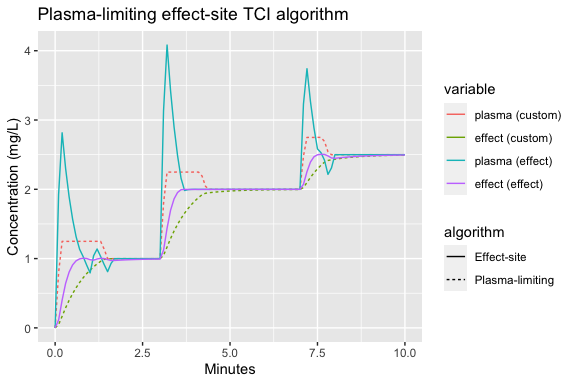# Custom PK models and TCI algorithms using the tci package

### Introduction

The tci package provides closed-form solutions for 1-, 2-, 3-, and 3-compartment with effect-site PK models based on solutions described and code provided in Abuhelwa, Foster, and Upton (2015). It does not, however, include an ordinary differential equation (ODE) solver as many other R packages do, including mrgsolve, PKPDsim, and RxODE. tci package functions can nonetheless be applied to models from these packages through the creation of user-defined PK functions.

### Custom PK models

Custom PK models based on ODEs or analytical solutions can be passed to pkmod objects through the pkfn argument. To illustrate this functionality, we consider the PK of the analgesic remifentanil. Remifentanil is an opioid derivative that is often administered intravenously to induce analgesia alongside propofol. Here, we consider the three-compartment PK model proposed by . Remifentanil is infused into a central compartment, representing the blood supply, and then circulated to two peripheral compartments, representing highly-perfused and scarcely-perfused organs and tissues. Remifentanil is then removed from all three-compartments with separate clearance rates. A diagram of the three-compartment model, reproduced from , is displayed in figure $$\ref{fig:Cascone_fig2}$$. The differential equations describing the remifentanyl model are given in equations $$\ref{eq:remif}$$.

\begin{equation}\label{eq:remif} \begin{aligned} V_1 \cdot \frac{dC_p}{dt} &= -Cl_1 \cdot C_1 + k_{21}\cdot V_2 \cdot C_2 + k_{31}\cdot C_3 \cdot V_3 + -[(k_{12}+k_{13}+k_{10})\cdot C_1] \cdot V_1 + k_R(t) \\ V_2 \cdot \frac{dC_2}{dt} &= k_{12} \cdot C_1 \cdot V_1 - k_{21} \cdot C_2 \cdot V_2 - Cl_2 \cdot C_2 \\ V_3 \cdot \frac{dC_3}{dt} &= k_{13} \cdot C_1 \cdot V_1 - k_{31} \cdot C_3 \cdot V_3 - Cl_3 \cdot C_3 \end{aligned} \end{equation}

To implement the ODE system in mrgsolve we initialize a model using mrgsolve::mcode with default parameter values.

library(tci)
library(mrgsolve) # implement ODE equation
library(xtable)   # printing tables
library(ggplot2)  # plotting results
library(reshape2) # melt function

form <- '
$PARAM V1 = 7.88, V2=23.9, V3=13.8, CL1=5, CL2=0.828, CL3=0.0784, k10 = 0.172, k12=0.373, k21=0.103, k13=0.0367, k31=0.0124$CMT A1 A2 A3
$ODE dxdt_A1 = k21*A2 + k31*A3 - (k12+k13+k10)*A1 - CL1/V1*A1; dxdt_A2 = k12*A1 - k21*A2 - CL2/V2*A2; dxdt_A3 = k13*A1 - k31*A3 - CL3/V3*A3; ' mrg_mod_remif <- mcode("remifentanil", form) For a custom model to be compatible with PK, it must take as arguments 1) a vector of time points, tm, 2) an numeric value describing a constant infusion rate, kR, 3) a vector of PK parameter values, pars, and 4) initial starting concentrations, init. Notably, init should be created with default values, as pkmod will use the initial values to determine the number of compartments in the model. pk_remif <- function(tm, kR, pars, init = c(0,0,0)){ # allow lowercase names names(pars) <- toupper(names(pars)) # store volume vols <- pars[c("V1","V2","V3")] A0 <- init*vols # initial amounts names(A0) <- c("A1","A2","A3") # names required by mrgsolve # pass parameters as list pars <- sapply(pars, as.list) # update parameters and initial values (as amounts) mrg_mod_remif <- update(mrg_mod_remif, param = pars, init = A0) # dosing regimen - mrgsolve function in terms of amount infused event <- ev(amt = kR*max(tm), time = 0, tinf = max(tm)) # simulate responses (skip tm=0 unless specified) dat <- mrgsim_q(x = mrg_mod_remif, # pk model data = event, # dosing event stime = tm) # evaluation times # skip tm=0 unless specified in tm dat <- dat@data[-1,] # return concentrations with compartments in rows and times in columns cons <- t(dat[,c("A1","A2","A3")]) / vols rownames(cons) <- colnames(cons) <- NULL return(cons) } We can now evaluate the remifentanil PK model as we would any of the internal PK functions. The optimized parameter values identified by , which we will use as an example, are reproduced in Table $$\ref{tab:Cascone-tab1}$$. % latex table generated in R 4.0.4 by xtable 1.8-4 package dose_remi <- inf_manual(inf_tms = 0, inf_rate = 60, duration = 20) pars_remif <- c(V1 = 7.88, V2=23.9, V3=13.8, CL1=5, CL2=0.828, CL3=0.0784, k10 = 0.172, k12=0.373, k21=0.103, k13=0.0367, k31=0.0124) mod_remif <- pkmod(pkfn = pk_remif, pars_pk = pars_remif) p1 <- predict(mod_remif, inf = dose_remi, tms = 0:80) ggplot(melt(data.frame(time = 0:80, p1), id = "time"), aes(x = time, y = value, color = variable)) + geom_line()### Custom TCI algorithms The tci package implements the Jacobs and Shafer algorithms plasma- and effect-site targeting algorithms, respectively (Jacobs 1990; Shafer and Gregg 1992). These algorithms aim to reach the target concentrations as quickly as possible without overshooting the target. There may, however, be situations in which the speed of target attainment is not the only goal. In these cases, a user may wish to specify a different TCI algorithm. An example of this is the algorithm proposed by Van Poucke, Bravo, and Shafer (2004) that limits the maximum percentage overshoot of the target in the central compartment. The motivation for this is that there may exist cases in which excessive plasma concentrations are associated with toxicity to the patient. Here, we construct a similar algorithm that limits the absolute, rather than the percentage, overshoot in the central compartment. In this example algorithm the user specifies a permissible amount of overshoot in the central compartment, , beyond the nominal target. At each step, the example TCI algorithm defines a maximum plasma concentration to equal the target effect-site concentration plus the permissible overshoot: . It then calculates the infusion required to reach or maintain over the subsequent ten seconds, . It then calculates the maximum effect-site concentration if is given, . If is less than the target concentration, can be administered without overshoot. If is greater than the target concentration, then targeting the effect-site directly will result in a maximum plasma concentration less than and the effect-site targeting algorithm is applied. The required arguments for a custom algorithm are 1) a single numeric value specifying the target concentration, Ct, 2) a pkmod object created by pkmod(), 3) a single numeric value specifying the infusion duration, dtm, and 4) additional arguments that are passed to update.pkmod at the beginning of the algorithm. Argument (4) is used to update starting concentrations when the algorithm is iteratively applied in inf_tci. tci_plasma_lim <- function(Ct, pkmod, dtm = 1/6, maxrt = 1200, lim_amt = 0.5, ecmpt = NULL, tmax_search = 20, cetol = 0.05, cptol = 0.1, ...){ pkmod <- update(pkmod,...) # if effect-site concentration is close to target, # switch to plasma targeting if(with(pkmod,(Ct - init[ecmpt]) / Ct < cetol & (Ct - init[pcmpt])/Ct <= cptol)) return(tci_plasma(Ct, pkmod = pkmod, dtm = dtm, maxrt = maxrt)) # maximum tolerable plasma concentration Cp_max <- Ct + lim_amt # infusion required to reach Cp_max pinf <- tci_plasma(Ct = Cp_max, pkmod = pkmod, dtm = dtm, maxrt = maxrt) # Administer dtm-minute infusion unit_inf <- inf_manual(inf_tms = 0, inf_rate = pinf, duration = dtm) # Calculate maximum effect-site concentration CeP <- function(tm) predict(pkmod, inf = unit_inf, tms = tm)[,pkmod$ecmpt]
Ce_max <- optimize(CeP, c(0,20), maximum = TRUE)$objective # if max Ce < Ct administer infusion to reach maximum target if(Ce_max <= Ct + cetol*Ct) infrt <- pinf else infrt <- tci_effect_only(Ct, pkmod, dtm, maxrt = maxrt) return(infrt) } We can now apply the algorithm directly to a pkmod object to calculate a single infusion rate. mod3ecpt <- pkmod(pars_pk = c(cl = 10, q2 = 2, q3 =20, v = 15, v2 = 30, v3 = 50, ke0 = 1.2)) tci_plasma_lim(Ct = 2, pkmod = mod3ecpt, lim_amt = 0.25) #>  240.0374 More usefully, however, we can pass the algorithm to inf_tci through the custom_alg argument and use it to calculate infusion rates required to reach a series of targets. # tci target concentrations tci_targets <- cbind(value = c(1,2,2.5,2.5), time = c(0,3,7,10)) # calculate infusion schedule using plasma-limiting algorithm plim_inf <- inf_tci(target_vals = c(1,2,2.5,2.5), target_tms = c(0,3,7,10), pkmod = mod3ecpt, custom_alg = tci_plasma_lim, lim_amt = 0.25) head(plim_inf) #> begin end inf_rate Ct c1_start c2_start c3_start c4_start #> [1,] 0.00000 0.16667 133.35413 1 0.00 0.000000000 0.00000000 0.0000000 #> [2,] 0.16667 0.33333 38.27734 1 1.25 0.007317989 0.04308491 0.1236035 #> [3,] 0.33333 0.50000 36.74880 1 1.25 0.021033000 0.12082833 0.3275202 #> [4,] 0.50000 0.66667 35.31726 1 1.25 0.034597490 0.19356382 0.4944899 #> [5,] 0.66667 0.83333 33.97649 1 1.25 0.048013056 0.26161398 0.6312089 #> [6,] 0.83333 1.00000 32.72067 1 1.25 0.061281283 0.32528061 0.7431596 #> c1_end c2_end c3_end c4_end #> [1,] 1.25 0.007317989 0.04308491 0.1236035 #> [2,] 1.25 0.021033000 0.12082833 0.3275202 #> [3,] 1.25 0.034597490 0.19356382 0.4944899 #> [4,] 1.25 0.048013056 0.26161398 0.6312089 #> [5,] 1.25 0.061281283 0.32528061 0.7431596 #> [6,] 1.25 0.074403741 0.38484609 0.8348308 For comparison, we calculate the infusion schedule associated with direct effect-site targeting. # effect-site targeting eff_inf <- inf_tci(target_vals = c(1,2,2.5,2.5), target_tms = c(0,3,7,10), pkmod = mod3ecpt, type = "effect") We now can use the infusion schedule in predict.pkmod or plot.pkmod methods # predict responses tms_pred <- seq(0,10,0.1) plim_pred <- predict(mod3ecpt, plim_inf, tms_pred) eff_pred <- predict(mod3ecpt, eff_inf, tms_pred) # plot results dat <- data.frame(time = tms_pred, plasma (custom) = plim_pred[,"c1"], effect (custom) = plim_pred[,"c4"], plasma (effect) = eff_pred[,"c1"], effect (effect) = eff_pred[,"c4"], check.names = FALSE) datm <- melt(dat, id = "time") datm$algorithm <- ifelse(datm\$variable %in% c("plasma (custom)","effect (custom)"),
"Plasma-limiting", "Effect-site")
ggplot(datm, aes(x = time, y = value, color = variable, linetype = algorithm)) +
geom_line() +
xlab("Minutes") +
ylab("Concentration (mg/L)") +
ggtitle(label = "Plasma-limiting effect-site TCI algorithm")